JEE  >  Integration for Physics

# Integration for Physics - Notes | Study Physics For JEE - JEE

 1 Crore+ students have signed up on EduRev. Have you?

Integration is an inverse process of differentiation.
It is the process of finding the function whose derivative is given.
Suppose F(x) is the derivative of the function f(x) w.r.t.x. Then we can write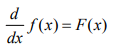Now, if we are given the derivative F(x) and we have to find the function f(x) then this can be done with the help of Integral Calculus.
The process of finding the function whose derivative is given is called integration.

Definition: If the derivative of a function f(x) is F(x) then f(x) is called the integral of F(x) with respect to x. The integration of a function can be written as ∫ F(x)dx = f (x). The function F(x) whose integral is f(x) is called Integrand.

Fundamental Formulae of Integration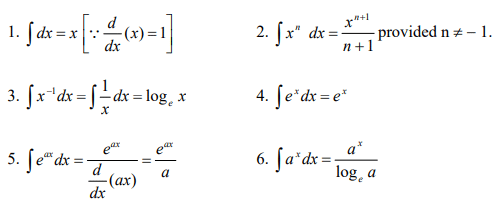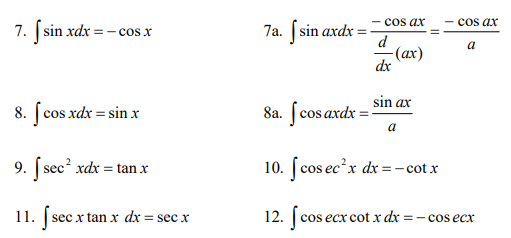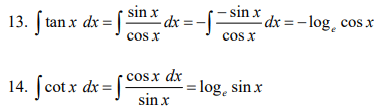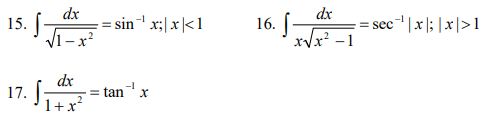Theorems of Integration

First Theorem: The integral of the product of a constant and a function is equal to the product of the constant and integral of the function.
i.e ∫ c u d x = c ∫ u d x , where c is constant.

Second Theorem: The integral of the sum or difference of a number of functions is given b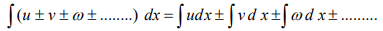The document Integration for Physics - Notes | Study Physics For JEE - JEE is a part of the JEE Course Physics For JEE.
All you need of JEE at this link: JEE

## Physics For JEE

257 videos|633 docs|256 tests
 Use Code STAYHOME200 and get INR 200 additional OFF

## Physics For JEE

257 videos|633 docs|256 tests

Track your progress, build streaks, highlight & save important lessons and more!

,

,

,

,

,

,

,

,

,

,

,

,

,

,

,

,

,

,

,

,

,

;